## Introduction

Quantum teleportation, the carrier-less transmission of quantum information by transferring a state from one quantum system to a remote one was described by Bennett et al.1 and soon after played an important role in photonic quantum computing2,3,4,5 as well as secure communication by means of quantum key distribution (e.g.6,7). The fragile nature of quantum systems and nearly omnipresent dissipative environments make it challenging to realize quantum teleportation experimentally. Bouwmeester et al.8 were the first to achieve quantum teleportation followed by many others for discrete-level quantum systems9,10,11,12,13,14,15 as well as with continuous variables16,17,18,19. In the case of discrete-level quantum systems so far only the state of the most simple quantum systems, i.e., two-level systems and therewith the smallest unit of quantum information (a “qubit”) could be teleported. A new teleportation scheme, proposed recently20, is capable to transmit the quantum information carried by an elementary excitation of a superposition of an arbitrary number d of co-propagating light modes (a photonic “qudit”). The teleportation of photonic qudits increases the quantum information sent per carrier photon. Currently, the low transmission rates are one of the bottlenecks of quantum communication as compared to its classical counterpart. However, the scheme proposed in20 requires to prepare d additional photons in a highly entangled state. Here we present an alternative scheme based on the transcription of the qudit encoded in a single photon to d qubits carried by light modes which propagate along different optical paths. Each qubit contains the quantum information about the excitation of a particular of the d original light modes and is teleported individually by means of an additional photon using quantum scissors.

Quantum scissors15,21,22,23,24 is a device to teleport only the vacuum and the single-photon component of a single-mode state (a so-called single-rail qubit), while it truncates (“cuts off”) higher photon-number components. In particular, if an input light mode c (cp. Fig. (1)) is prepared in a superposition of states with different photon numbers, quantum scissors projects its vacuum and single photon component to an ouput mode b:where |nc(b) represents a so-called Fock state with n = 0, 1, 2 … photons in light mode c (b) and the coefficients αn are the corresponding probability amplitudes. This process occurs upon conditioning on a single-photon detection with the probability given by the square of the norm of the final state |χ′〉b, i.e, (|α0|2 + |α1|2)/4. However, this probability can be doubled by conditioning on one of two possible single-photon detections (cp. Methods). The working principle of quantum scissors is explained in the caption of Fig. (1).

On the other hand, if the input state in mode c consists already of a single-rail qubit, i.e. |χ〉c = (α0 |0〉c + α1 |1〉c), it is transferred according to transformation (1) without truncation and hence teleported, to mode b. A generalization of quantum scissors which cuts off all state components with a number of d or more photons and thus teleports multi-photon states of the form(“single-rail qudits”) can be achieved using multiports and d − 1 additional input photons25,26. However, the encoding of an arbitrary superposition |χ〉c of multiple photon-number Fock states is in practice difficult and requires non-linear optical media leading to small efficiencies27,28,29.

Moreover, one can teleport n single-rail qubits simultaneously, provided they are stored in light modes which propagate on different paths, by applying n quantum scissor setups in parallel, one for each single-rail qubit. Obviously, the simultaneous teleportation works if the single-rail qubits in the individual modes are not correlated. But note, that also the state of n entangled qubits can be teleported in this way.

## Results

This feature of quantum scissors can be exploited to teleport a qudit encoded into a single photon which is shared by d spatial modes of paraxial light. For this purpose the d-level state of the photon is transcribed into d single-rail qubits carried by light modes propagating along different paths with the help of a mode sorter. Such a device has the task to transfer orthogonal light modes within a single light beam to different optical paths, similar to a polarizing beam splitter, which conveys light with horizontal and vertical polarization to orthogonal paths. For example, consider a single-photon state |χ〉 given by an elementary excitation of a superposition of d paraxial Laguerre-Gauss modes LGl,p = 0 corresponding to different valuesof orbital angular momentum (OAM)30 which co-propagate along an optical path o, i.e,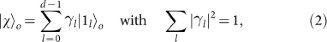where |1l〉 denotes the state of a single photon with OAM. We spatially separate the OAM modes by diverting them into different optical paths cl depending on their OAM value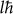with the help of an OAM mode sorter31,32. For d = 3 this transformation reads:where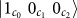represents a single photon with OAM quantum number l = 0 in path c0 and no photon in all other paths (accordingly for the remaining terms). The single photon states are conveniently expressed by the creation operatorsacting on the global vacuum state |0〉, cp. the right-hand side of (3). This transformation transcribes the state of a qudit into d entangled single-rail qubits. After the transcription the ith qubit contains the quantum information about whether the corresponding OAM mode l = i of the photonic qudit was occupied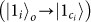or not.

Now each single-rail qubit can be teleported individually (cp. Fig. (2)) using quantum scissors. This is accomplished as follows: each of the d spatial modes are inserted into d independent quantum scissors setups (see Fig. (3) for d = 2). There are thus d input modes ci and d output modes bi with i = 0, 1 … d − 1 to carry the quantum information. In addition, the quantum scissors require a total of d single photons entered separately in modes ai. Upon conditioning on the detection of a single photon in each of the quantum scissor devices (success probability 1/2d with ideal detectors, for non-unit detection efficiencies see Methods) the state |χ〉 carried by the input modes ci is transferred to the output modes bi (cp. Methods):Since the mode bi in the ith quantum scissors device originates from the reflection of mode ai both are identical except for their propagation direction and they should carry the same OAM value as input mode ci, the state of which is supposed to be transferred to bi. Hence, the photon entering in mode ai should be prepared with OAM value.

However, as shown under Methods, this is not necessary if the modes bi are transformed into the appropriate OAM mode after the state transfer. In fact, preparing the additional photons in a different system of basis modes enables a transcription of the quantum information stored in a specific basis (here OAM modes) in the input modes of the quantum scissors devices to another basis (for example Hermite Gaussian modes33) in its output modes. By such a transcription any unitary gate acting on the Hilbert space of the qudit can be realized, however only with limited success probability which is determined by the quantum scissors involved.

After successful teleportation by the quantum scissors we can convert the entangled d single-rail qubits back to the original d-mode OAM state (2) with the help of a mixer which is a sorter run in reverse. This completes the teleportation of a photonic qudit (cp. Fig. (2)). In order to realize an additional unitary qudit-gate (see above) together with the teleportation, the mixer has to map the new basis modes in the outputs of the d quantum scissors into a single beam, i.e., it must be a reverse sorter for these particular modes, which exists for example for Hermite Gaussian modes33.

## Discussion

In this article we have presented a scheme to teleport a photonic qudit carried by OAM modes. The scheme requires linear optical devices, OAM mode sorters as well as single-photon sources and photon-number resolving detectors. The essential step is to transcribe the state of the qudit to d single-rail qubits by means of a mode sorter and to teleport the qubits individually by quantum scissors. In as far as such sorter devices can be designed for other light modes, for example Hermite-Gaussian modes33, the proposed teleportation scheme is universal and can be implemented with any system of basis modes. Using quantum scissors a single-rail qubit can be teleported with success probability 1/2, therefore, the success probability to teleport d single-rail qubits and thus the encoded qudit amounts to 1/2d.

In principle, the probability to teleport a single-rail qubit can be increased to N/(N + 1) by employing N additional entangled photons and a balanced multiport with N + 1 inputs and outputs as described by Knill et al.4 instead of one additional photon and a balanced beam splitter in each of the quantum scissors setups. This leads to a success probability for the qudit teleportation of (N/(N + 1))d but requires d highly entangled N-photon states, which can be prepared probabilistically offline4,34.

On the other hand, the encoding of one qudit into d two-level systems (such as single-rail qubits) represents an inefficient use of storage capacity. The amount of quantum information present in a single qudit actually corresponds to log2 d qubit units of quantum information and could thus be stored efficiently in log2 d single-rail qubits. Given a scheme which is able to transcribe the initial photonic qudit into log2 d single-rail qubits, a subsequent teleportation could be achieved by means of log2 d quantum scissors with a success probability of. This would mean an exponential decrease of the resources needed to teleport a qudit.

Alternatively, using the transcription based on a OAM mode sorter, as described above, the present scheme allows, instead of a single qudit, to teleport d single-rail qubits encoded in co-propagating OAM modes, with the same resources as before. This corresponds to an exponential increase of quantum information sent per use of the teleportation protocol. However, the preparation and manipulation of single-rail qubits seems problematic compared to qudits carried by single-photon states of OAM modes, which can be prepared, transformed and measured with standard techniques35. For single-rail qubits, general deterministic single- and two-qubit gates are not available36. Moreover, the vacuum component makes state tomography of single-rail qubits difficult.

The present scheme has certain advantages as well as disadvantages over a recently proposed alternative teleportation method20. Unlike the latter, it does not require highly sensitive multipartite entangled states to perform the quantum teleportation. On the other hand, the alternative method yields a greater success probability of 1/d2 for qudit teleportation and requires less additional photons. Remarkably, it yields for the joint teleportation of the state of many photons the same maximal teleportation rate as quantum scissors for single rail qubits, namely one qubit per additional photon. However, by improving the transcription efficiency one could overcome these drawbacks of the present scheme.

## Methods

In the following we show that d quantum scissors enable a transfer of the state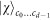obtained after the transformation (3) to output modes b0bd − 1 (4). The state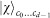along with d photons at ports ai constitute the initial state entering the d quantum scissors (cp. Fig. (3) for d = 2)where we have introduced the creation operatorsto denote single photons in the modes ai. Each quantum scissors device contains two 50:50 beam splitters, cp. Fig. 1. The first beam splitter BS1 of the ith device distributes the incoming photon in mode ai equally over both modes, ai and bi, represented by the transformation rule in terms of the corresponding creation operators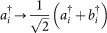. Also the action of the second beam splitter BS2 in each quantum scissors device is conveniently described by similar rules: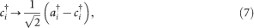Consecutive application of these transformations for beam splitters BS1 and BS2 for all quantum scissors i to the initial state (5) yields the total state change: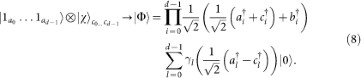The second and final step to complete the state transfer by means of quantum scissors provides a photon-number measurement in modes ai and ci conditioned on the detection of a single photon in ai and vacuum in ci, cp. Fig. 1. Since there are a total of d + 1 photons in the system, a detection of one photon in each of the d modes ai and zero photons in the modes ci results in a single photon in one of the modes bi according to photon-number conservation. The measurement projects onto those components of state |Φ〉 in Eq. (8) which allow for such a detection event:Therefore the state of light in the output modes b0bd − 1 of the quantum scissors reads:which is the state initially carried by the input modes cl, cf. (5).

Please note, that a teleportation of the initial state carried by the input modes ci onto different output modes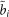can also be achieved:For this purpose, photons of modes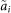corresponding to the targeted modes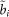are inserted into the ports ai, together with the initial state |χ〉 in modes ci;The consecutive actions of the beam splitters BS1 and BS2 in the quantum scissor devices, given respectively by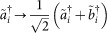and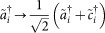together with (7), transform state (12) intoThe only components of state |Ψ〉 that can contribute to a coincidence detection of a single photon by the detectors D1 (cp. Fig. 1) in each of the d quantum scissors devices are given by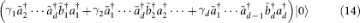If we further assume that each detectors D1 absorbs a single photon in the detection process without distinguishing between both kinds of photons24, ai and, then the remaining state is given as claimed byThe detection event indicating successful teleportation occurs for ideal detectors with probability 1/22d which is obtained from a normalization factor in projection (9). However, the success probability can be increased by considering other detection events. For example, if one photon is detected in mode cj instead of mode aj, as well as one photon in each of the remaining modes ai, the state of b collapses into:where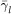is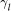for lj and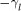for l = j. The minus sign can be compensated by applying a π-phase shift to mode bj which causes the state change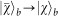. Hence, it does not matter whether the detectors in modes aj or cj register a single photon count as long as there is only one count in each quantum scissors setup. Thus there are 2d detection events corresponding to successful teleportation, which increases the probability of success to 2d/22d = 1/2d.

The success probability of the teleportation scheme depends on the efficiencies of the detectors used with the quantum scissors. For detectors which count a single photon with probability (i.e., efficiency) η < 1 the success probability of the scheme reduces to (η/2)d. Moreover, a restricted detection efficiency can induce the false identification of a two-photon detection event as a single-photon count with probability 2η(1 − η). Such a mistaken identification in one of the quantum scissor set-ups together with single photon counts in the remaining ones leads to vacuum in the output modes, while the detectors seemingly announce a successful teleportation. The probability for such false announcement equals η(1 − η) and can be calculated from the probability to obtain a two-photon detection event with ideal detectors which amounts to 1/2, independent of the number of quantum scissor setups (cp. Eq. (8)), multiplied with the probability for a false identificaton due to the non-unit detection efficiency. Therefore, the teleportation fidelity defined as the overlap between the input and the output state of the teleportation scheme decreases from f = 1 with ideal detectors to f = 1 − η(1 − η) for detectors with efficiency η.